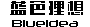您的位置：　首页 > 技术文档 > 网页制作 > 表单验证 Validator v1.05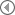JavaScript实用的一些技巧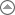回到列表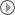Web标准发展建议与实例表单验证 Validator v1.05
 作者：我佛山人　时间： 2004-11-26　文档类型：原创　来自：蓝色理想表单的验证一直是网页设计者头痛的问题，表单验证类 Validator就是为解决这个问题而写的，旨在使设计者从纷繁复杂的表单验证中解放出来，把精力集中于网页的设计和功能上的改进上。 Validator是基于JavaScript技术的伪静态类和对象的自定义属性，可以对网页中的表单项输入进行相应的验证，允许同一页面中同时验证多个表单，熟悉接口之后也可以对特定的表单项甚至仅仅是某个字符串进行验证。因为是伪静态类，所以在调用时不需要实例化，直接以"类名+.语法+属性或方法名"来调用。此外，Validator还提供3种不同的错误提示模式，以满足不同的需要。 Validator目前可实现的验证类型有： [JavaScript] 版Validator目前可实现的验证类型有： 1.是否为空；2.中文字符；3.双字节字符4.英文；5.数字；6.整数；7.实数；8.Email地址；9.使用HTTP协议的网址；10.电话号码；11.货币；12.手机号码；13.邮政编码；14.身份证号码(1.05增强)；15.QQ号码；16.日期；17.符合安全规则的密码；18.某项的重复值；19.两数的关系比较；20.判断输入值是否在(n, m)区间；21.输入字符长度限制(可按字节比较)；22.对于具有相同名称的单选按钮的选中判断；23.限制具有相同名称的多选按钮的选中数目；24.自定义的正则表达式验证； 25.文件上传格式过滤(1.04)运行环境(客户端)： 在Windows Server 2003下用IE6.0+SP1和Mozilla Firefox 1.0测试通过；在Lunix RedHat 9下的Netscape测试通过； 对于客户端的表单验证，这个基于JavaScript编写的Validator基本上都可以满足，具体可以下载CHM文件：Validator.CHM下载 示例： 运行代码框 表单验证类 Validator v1.05

ID：

QQ：

[Ctrl+A 全部选择 提示：你可先修改部分代码，再按运行] 更新历史： 1.01修正对12月份的日期验证(感谢flylg999) 1.03修正Range验证类型时将数字当字符串比较的bug(感谢cncom和xtlhnhbb)修正日期验证(感谢Papsam)增加Username验证类型增加对Phone验证类型时支持分机号 1.04增加文件格式的过滤，用于上传时限制上传的文件格式 1.05增强对身份证号码的验证 [PHP]版代码拷贝框 表单验证类 Validator for PHP β

QQ：

submit = \$submit_name; \$this->error_mode = \$mode; \$this->error_no = 1; } function Validate(\$arr){ if(! isset(\$_POST[\$this->submit])) return false; \$this->error_mode = \$_POST["emode"]; echo ""; if(is_array(\$arr)){ \$len = count(\$arr); for(\$i = 0; \$i < \$len; \$i++){ \$this->is_valid(\$arr[\$i]); } } if(\$this->error_no > 1) \$this->display_error(); } function is_valid(\$str){ \$str = split(",", \$str); if(count(\$str) < 3) return false; \$name = trim(\$str); \$message = trim(\$str); \$data_type = trim(\$str); \$value = trim(\$_POST[\$name]); switch(\$data_type){ case "compare" : break; case "range" : break; case "repeat" : break; default : \$method = "is_".\$data_type; if(!\$this->\$method(\$value)) \$this->add_error(\$name, \$message); break; } } function add_error(\$name, \$message){ \$this->error_item .= "," . \$name; \$this->error_message .= "," . \$this->error_no . ":" . \$message; \$this->error_no ++; } function display_error(){ \$this->error_item = ereg_replace("^,+", "", \$this->error_item); \$this->error_message = ereg_replace("^,+", "", \$this->error_message); switch(\$this->error_mode){ case 4 : \$info = "以下原因导致提交失败：\t\t\t\t,"; echo ""; //print >>>end; break; case 5 : echo "输入有错误：
• " . ereg_replace( "\b\d+:", "",join("
• ", split(",", \$this->error_message))) . "
"; echo "

]+>([\s\S]+)<\/form>[\s\S]*" Str = .Replace(Str, "\$1") .Global = True .MultiLine = True .Pattern = "<\/?(?!input|textarea|select)[^>]*>" Str = .Replace(Str, "") .Pattern = "^.*(<(?=input|textarea|select)[^>]*>).*\$" Str = .Replace(Str, "\$1") .Pattern = "([\r\n]+|^\s*)(?=<)" Str = .Replace(Str, "") While Test("dataType=([""\'])([^""\'>]+)\1", Str) .MultiLine = False .Pattern = "^([^\n]+)\n([\s\S]*)\$" itemString = .Replace(Str, "\$1") Str = .Replace(Str, "\$2") .Pattern = "(name|dataType|to1|min|max|msg|require|regexp|format)=([""\'])([^""\'>]+)\2" Dim Matches : Set Matches = .Execute(itemString) Dim Match, RetStr : RetStr = "" For Each Match in Matches RetStr = RetStr & Match.Value & " : " Next Call IsValid(Replace(Replace(Replace(RetStr, " : \$", ""), "to=", "toObj="), """Require""", """NotEmpty""")) Wend .IgnoreCase = bI .Global = bG .MultiLine = bM End With End Sub Private Sub IsValid(ByVal Str) Dim name, msg, dataType, toObj, min, max, require, regexp, format min = 1 : max = 100 : require = "true" : format = "YMD" Execute Str Dim PostValue : PostValue = Request(name) Dim Fun IF require = "false" AND PostValue = "" Then Exit Sub IF Dic.Exists(dataType) Then Fun = Dic.Item(dataType) Else Fun = "Is" & dataType &"( PostValue )" End IF IF Not Eval(Fun) Then Call AddError(name,msg) End Sub Private Sub DisplayError() ErrorItem = Replace(ErrorItem, "^(" & Separator & ")", "") ErrorMessage = Replace(ErrorMessage, "^(" & Separator & ")", "") Select Case ErrorMode Case 4 ErrorMessage = Join(Split(ErrorMessage, Separator), "
• ") Response.Clear Response.Write "
输入有错误：
• " & Replace(ErrorMessage, "\b\d+:", "") & "
" Response.Write "
返回重填
" Response.End Case Else Response.Write("") End Select End Sub Public Function IsEmail(ByVal Str) IsEmail = Test("^\w+([-+.]\w+)*@\w+([-.]\w+)*\.\w+([-.]\w+)*\$", Str) End Function Public Function IsUrl(ByVal Str) IsUrl = Test("^http:\/\/[A-Za-z0-9]+\.[A-Za-z0-9]+[\/=\?%\-&_~`@[\]\':+!]*([^<>""])*\$", Str) End Function Public Function IsNum(ByVal Str) IsNum= Test("^\d+\$", Str) End Function Public Function IsQQ(ByVal Str) IsQQ = Test("^[1-9]\d{4,8}\$", Str) End Function Public Function IsZip(ByVal Str) IsZip = Test("^[1-9]\d{5}\$", Str) End Function Public Function IsIdCard(ByVal Str) IsIdCard = Test("^\d{15}(\d{2}[A-Za-z0-9])?\$", Str) End Function Public Function IsChinese(ByVal Str) IsChinese = Test("^[\u0391-\uFFE5]+\$", Str) End Function Public Function IsEnglish(ByVal Str) IsEnglish = Test("^[A-Za-z]+\$", Str) End Function Public Function IsMobile(ByVal Str) IsMobile = Test("^((\(\d{3}\))|(\d{3}\-))?13\d{9}\$", Str) End Function Public Function IsPhone(ByVal Str) IsPhone = Test("^((\(\d{3}\))|(\d{3}\-))?(\(0\d{2,3}\)|0\d{2,3}-)?[1-9]\d{6,7}\$", Str) End Function Public Function IsSafe(ByVal Str) IsSafe = (Test("^(([A-Z]*|[a-z]*|\d*|[-_\~!@#\\$%\^&\*\.\(\)\[\]\{\}<>\?\\\/\'\""]*)|.{0,5})\$|\s", Str) = False) End Function Public Function IsNotEmpty(ByVal Str) IsNotEmpty = LenB(Str) > 0 End Function Public Function IsDateFormat(ByVal Str, ByVal Format) IF Not IsDate(Str) Then IsDateFormat = False Exit Function End IF IF Format = "YMD" Then IsDateFormat = Test("^((\d{4})|(\d{2}))([-./])(\d{1,2})\4(\d{1,2})\$", Str) Else IsDateFormat = Test("^(\d{1,2})([-./])(\d{1,2})\\2((\d{4})|(\d{2}))\$", Str) End IF End Function Public Function IsEqual(ByVal Src, ByVal Tar) IsEqual = (Src = Tar) End Function Public Function Compare(ByVal Op1, ByVal Operator, ByVal Op2) Compare = False IF Dic.Exists(Operator) Then Compare = Eval(Dic.Item(Operator)) Elseif IsNotEmpty(Op1) Then Compare = Eval(Op1 & Operator & Op2 ) End IF End Function Public Function Range(ByVal Src, ByVal Min, ByVal Max) Min = CInt(Min) : Max = CInt(Max) Range = (Min < Src And Src < Max) End Function Public Function Group(ByVal Src, ByVal Min, ByVal Max) Min = CInt(Min) : Max = CInt(Max) Dim Num : Num = UBound(Split(Src, ",")) + 1 Group = Range(Num, Min - 1, Max + 1) End Function Public Function Custom(ByVal Str, ByVal Reg) Custom = Test(Reg, Str) End Function Public Function Limit(ByVal Str, ByVal Min, ByVal Max) Min = CInt(Min) : Max = CInt(Max) Dim L : L = Len(Str) Limit = (Min <= L And L <= Max) End Function Public Function LimitB(ByVal Str, ByVal Min, ByVal Max) Min = CInt(Min) : Max = CInt(Max) Dim L : L =bLen(Str) LimitB = (Min <= L And L <= Max) End Function Private Function Test(ByVal Pattern, ByVal Str) Re.Pattern = Pattern Test = Re.Test(Str) End Function Public Function bLen(ByVal Str) bLen = Len(Replace(Str, "[^\x00-\xFF]", "..")) End Function Private Function Replace(ByVal Str, ByVal Pattern, ByVal ReStr) Re.Pattern = Pattern Replace = Re.Replace(Str, ReStr) End Function Private Function B2S(ByVal iStr) Dim reVal : reVal= "" Dim i, Code, nCode For i = 1 to LenB(iStr) Code = AscB(MidB(iStr, i, 1)) IF Code < &h80 Then reVal = reVal & Chr(Code) Else nCode = AscB(MidB(iStr, i+1, 1)) reVal = reVal & Chr(CLng(Code) * &h100 + CInt(nCode)) i = i + 1 End IF Next B2S = reVal End Function Private Sub AddError(ByVal Name, ByVal Message) ErrorItem = ErrorItem & Separator & Name ErrorMessage = ErrorMessage & Separator & ErrorNo & ":" & Message ErrorNo = ErrorNo + 1 End Sub Public Function IsValidPost() Dim Url1 : Url1 = Cstr(Request.ServerVariables("HTTP_REFERER")) Dim Url2 : Url2 = Cstr(Request.ServerVariables("SERVER_NAME")) IsValidPost = (Mid(Url1, 8, Len(Url2)) = Url2) End Function Public Property Let Mode(ByVal Val) ErrorMode = CInt(Val) End Property Public Property Let Form(ByVal Val) IF IsNumeric(Val) Then FormIndex = Val Else FormName = Val End IF End Property Public Property Let Path(ByVal Val) IF Test("^[A-Za-z]:\\\w+\$", Val) Then FilePath = Val Else FilePath = Server.MapPath(Val) End IF End Property Public Property Let Method(ByVal Val) GetMethod = Val End Property End Class %> 表单验证类 Validator v1.0
• 真实姓名：
英文名：
主页：
密码：
重复：
信箱：
信箱：
QQ：
身份证：
年龄：
年龄1：
电话：
手机：
生日：
邮政编码：
邮政编码：
操作系统：
所在省份：广东陕西浙江江西
爱好：运动上网听音乐看书
自我介绍：
自传：
<% Dim V : Set V = New Validator V.Mode = 3 V.Method = "XMLHTTP" V.Validate() Set V = Nothing %> [Ctrl+A 全部选择 然后拷贝] 出处：蓝色理想 责任编辑：红色黑客 ◎进入论坛网页制作、网站综合版块参加讨论相关文章 更多相关链接在 DW 中插入 Flash 的参数详解用js封装的时间设置器JavaScript 和 Flash 的通信UBB 转换函数演示用 onerror 获取错误信息作者文章表单验证 Validator v1.05Dreamweaver MX 2004打造留言本Dreamweaver MX 2004 试用心得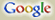全网 本站 论坛
 热门搜索：CSS Fireworks 设计比赛 网页制作 web标准 用户体验 UE photoshop Dreamweaver Studio8 Flash 手绘 CG站点最新 站点最新列表周大福“敬•自然”设计大赛开启国际体验设计大会7月将在京举行中国国防科技信息中心标志征集云计算如何让安全问题可控云计算是多数企业唯一拥抱互联网的机会阿里行云云手机年终巨献，送礼标配299起阿里巴巴CTO王坚的"云和互联网观"1499元买真八核 云OS双蛋大促首届COCO桌面手机主题设计大赛栏目最新 栏目最新列表浅谈JavaScript编程语言的编码规范如何在illustrator中绘制台历Ps简单绘制一个可爱的铅笔图标数据同步算法研究用ps作简单的作品展示页面CSS定位机制之一:普通流25个最佳最闪亮的Eclipse开发项目Illustrator中制作针线缝制文字效果Photoshop制作印刷凹凸字体VS2010中创建自定义SQL Rule分享按钮 蓝色理想版权申明：除部分特别声明不要转载，或者授权我站独家播发的文章外，大家可以自由转载我站点的原创文章，但原作者和来自我站的链接必须保留（非我站原创的，按照原来自一节，自行链接）。文章版权归我站和作者共有。 转载要求：转载之图片、文件，链接请不要盗链到本站，且不准打上各自站点的水印，亦不能抹去我站点水印。 特别注意：本站所提供的摄影照片，插画，设计作品，如需使用，请与原作者联系，版权归原作者所有，文章若有侵犯作者版权，请与我们联系，我们将立即删除修改。您的评论
用户名： 　口令：
说明：输入正确的用户名和密码才能参与评论。如果您不是本站会员，你可以注册 为本站会员。
注意：文章中的链接、内容等需要修改的错误，请用报告错误，以利文档及时修改。
不评分 1 2 3 4 5
注意：请不要在评论中含与内容无关的广告链接，违者封ID
请您注意：
・不良评论请用报告管理员，以利管理员及时删除。
・尊重网上道德，遵守中华人民共和国的各项有关法律法规
・承担一切因您的行为而直接或间接导致的民事或刑事法律责任
・本站评论管理人员有权保留或删除其管辖评论中的任意内容
・您在本站发表的作品，本站有权在网站内转载或引用
・参与本评论即表明您已经阅读并接受上述条款专业书推荐 更多内容网站可用性测试及优化指南《写给大家看的色彩书1》《跟我去香港》众妙之门―网站UI 设计之道《Flex 4.0 RIA开发宝典》《赢在设计》犀利开发―jQuery内核详解与实践作品集 更多内容